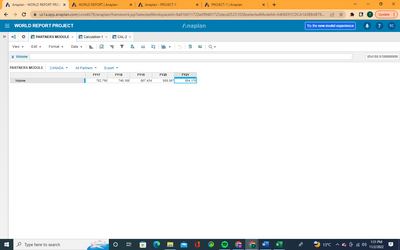# FormulaHello,

Quick question: How can I calculate the Volume between FY17 and F21 in the other module? For example, the difference between two years.
Note: Just Volume is a line item.

Thanks,

MarkTagged:

•Hi @Markcol

I see there are 4 dimensions (including time) in the current module. Understanding as much as possible from your description and the image, I assume you want to select one value from each dimension and then you want to calculate the difference between the volume.

Here is my idea:-

• Create one dimensionless module, add two line items for each dimension
• Dimension 1.1, Dimension 1.2, formatted by Dimension 1
• Dimension 2.1, Dimension 2.2, formatted by Dimension 2
• Dimension 3.1, Dimension 3.2, formatted by Dimension 3
• Period 1, Period 2,  formatted by period (Year)
• Volume 1, Volume 2, Number formatted. To store the values corresponding to other dimension
• Difference, number formatted. Formula:- Volume 1 - Volume 2
• Formula of Volume line items :- "The Source Volume line item"[Lookup: Dimension1.1, Lookup:Dimension 2.1, Lookup: Dimension 3.1, Lookup: Period 1]. Reference the other line items in Volume 2's formula.
• You'll have to manually select the values from list drop downs.

I hope it solves your problem.

Thanks!

-Shubham

•Hi @ShubhamCh,

Thank you very much for your detailed solution. I’ll try the solution.

All the best,

Mark